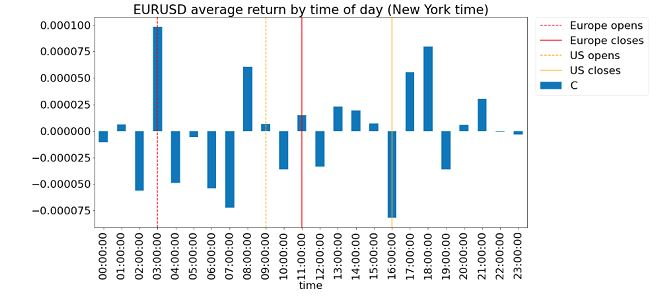## Sabtu, 03 Maret 2012

### Hidden Markov model applied to FX prediction

I read with interest an older paper "Can Markov Switching Models Predict Excess Foreign Exchange Returns?" by Dueker and Neely of the Federal Reserve Bank of St. Louis. I have a fondness for hidden Markov models because of its great success in speech recognition applications, but I confess that I have never been able to create a HMM model that outperforms simple technical indicators. I blame that both on my own lack of creativity as well as the fact that HMM tend to have too many parameters that need to be fitted to historical data, which makes it vulnerable to data snooping bias. Hence I approached this paper with the great hope that experts can teach me how to apply HMM properly to finance.

The objective of the model is simple: to predict the excess return of an exchange rate over an 8-day period. (Excess return in this context is measured by the % change in the exchange rate minus the interest rate differential  between the base and quote currencies of the currency pair.) If the expected excess return is higher than a threshold (called "filter" in the paper), then go long. If it is lower than another threshold, go short. Even though the prediction is on a 8-day return, the trading decision is made daily.

The excess return is assumed to have a 3-parameter student-t distribution. The 3 parameters are the mean, the degree of freedom, and the scale. The scale parameter (which controls the variance) can switch between a high and low value based on a Markov model. The degree of freedom (which controls the kurtosis, a.k.a. "thickness of the tails") can also switch between 2 values based on another Markov model. The mean is linearly dependent on the values assumed by the degree of freedom and the scale as well as another Markov variable that switches between 2 values. Hence the mean can assume 8 distinct values. The 3 Markov models are independent. The student-t distribution is more appropriate for the modelling financial returns than normal distribution because of the allowance for heavy tails. The authors also believe that this model captures the switch between periods of high and low volatility, with the consequent change of preference (=different mean returns) for "safe" versus "risky" currencies, a phenomenon well-demonstrated in the period between August 2011 to January 2012.

The parameters of the Markov models and the student-t distributions are estimated in the in-sample period (1974-1981) for each currency pair in order to minimize the cumulative deviation of the excess returns from zero. There are a total of 14 parameters to be so estimated. After these estimations, we have to also estimate the 2 trading thresholds by maximizing the in-sample return of the trading strategy, assuming a transaction costs of 10 basis point per trade.

With this large number (16 in total) of parameters, I dread to see the out-of-sample (1982-2005) results. Amazing, these are far better than I expected: the annualized returns range from 1.1% to 7.5% for 4 major currency pairs. The Sharpe ratios are not as impressive: they range from 0.11 to 0.71. Of course, when  researchers report out-of-sample results, one should take that with a grain of salt. If the out-of-sample results weren't good, they wouldn't be reporting them, and they would have kept changing the underlying model until good "out-of-sample" results are obtained! So it is really up to us to implement this model, apply it to data after 2005 and to more currency pairs, to find out if there is really something here. In fact, this is the reason why I prefer to read older papers - to allow for the possibility of true out-of-sample tests immediately.

What do you think can be done to improve this model? I suspect that as a first step, one can see whether the estimated Markov states correspond reasonably to what traders think of as risk-on vs risk-off regimes. If they do, then regardless of the usage of this model as a signal generator, it can at least generate good risk indicators. If not, then maybe the hidden Markov model need to be replaced with a Markov model that is conditioned on observable indicators.